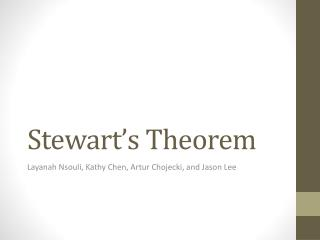# Stewart’s Theorem - PowerPoint PPT PresentationDownload PresentationStewart’s Theorem

Stewart’s TheoremDownload Presentation## Stewart’s Theorem

- - - - - - - - - - - - - - - - - - - - - - - - - - - E N D - - - - - - - - - - - - - - - - - - - - - - - - - - -
##### Presentation Transcript

1. Stewart’s Theorem Layanah Nsouli, Kathy Chen, ArturChojecki, and Jason Lee

2. Stewart’s Theorem

3. Stewart’s Theorem Variables • Given ∆ ABC • Sides a b c are opposite the vertices respectively • CP is drawn, it is called the cevian • AP is equal to m and PB is equal to n ( m + n = c )

4. Stewart’s Theorem Explained Given a triangle , let cevian CP be drawn. Then label AP as m and PB as n. Then label the entire side AB as c. Now, Stewart’s Theorem states that ma2 + nb2 = (m+n) PC2 + mn2 + nm2. So, you would plug in then values that were given to you already in the formula, and then solve it for whatever is missing.

5. Uses • This theorem is mainly used in geometric proof’s in order to prove that triangles are similar. When doing the proof, you use the parts of the formula as your reasons. • You are usually trying to find the measure of the cevian. • So, you plug in the variables that are given to you, and solve the equation step by step like explained above.

6. Example #1 In triangle ABC, AB=4, AC=6, AD=5 where D is the midpoint of BC. Determine BC.

7. Example #2 In triangle ABC, AC=12, AD=10, and AB=8. Point D is a midpoint of line CB. Determine the length of BD.

8. Example # 3 In triangle ABC, AC=10, CD= , and CB=20. Determine the length of AB to the nearest whole number. [Not drawn to Scale]